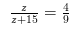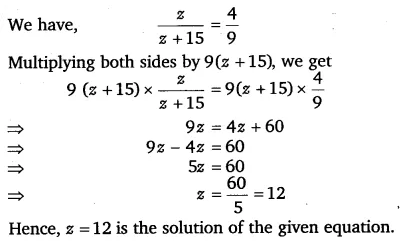# Solve the following equations: z/z+15 = 4/9

Solve the following equations: